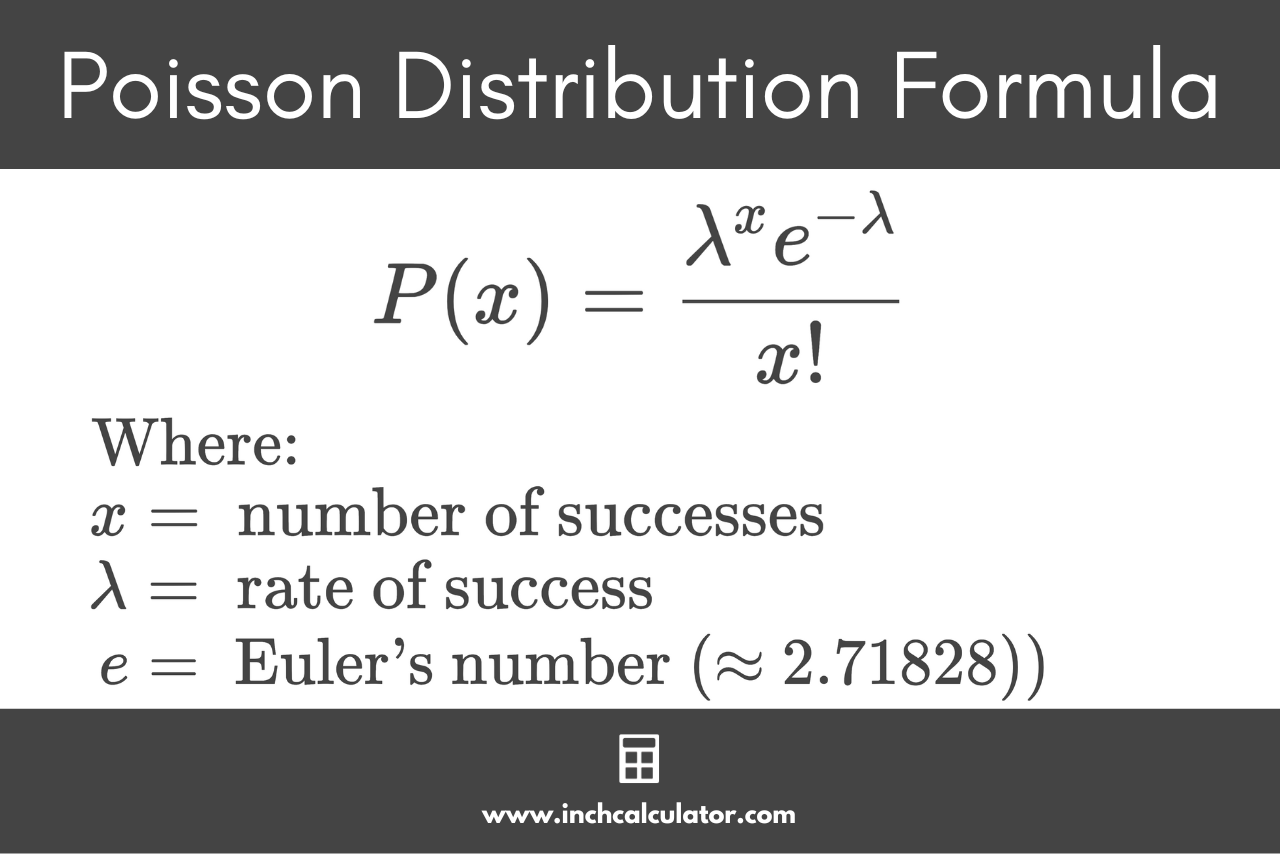# Poisson Distribution Calculator

Enter the average rate of success and the number of occurrences in the calculator below to find the probabilities of an event occurring using the Poisson distribution.

## Probabilities:

### Steps to Solve

#### Probability Mass Function

f(x; λ) = P(X = x) = λx × e / x!

#### Substitute Values in the Formula and Solve

The solution will be shown here after you enter values in the form above

Learn how we calculated this below

## What is a Poisson Distribution?

In statistics, the Poisson distribution is a probability distribution that describes the probability of an event occurring a specified number of times given an average rate of success. This is known as the Poisson probability.

In other words, the Poisson distribution estimates the likelihood of a specific number of occurrences of an event happening within a period of time.

The Poisson distribution is a probability distribution, but unlike a normal distribution, it is a discrete function that can only take non-negative whole numbers. Fractions or decimal values are not supported.

The Poisson distribution estimates the probability of independent events occurring, and the probability of each event is constant.

Unlike a binomial distribution which counts discrete occurrences among discrete trials, a Poisson distribution counts discrete occurrences among a continuous domain.

## How to Calculate a Poisson Probability

You can find the probability of events occurring using the Poisson distribution using the probability mass function. In order to use the function described by the formula below, you need to know the average rate of success, or the average number of times the event occurs.

### Poisson Distribution Formula

The probability of a specific number of occurrences of an event is described by the following formula:

P(x) = λxe / x!

Where:

• x is the number of occurrences (Poisson random variable)
• λ is the rate of success (Greek letter lambda)
• e ≈ 2.71828 (Euler’s number)
• both x and λ are non-negative integers

Thus, the probability of an event occurring x number of times is equal to the average rate of success λ to the power of x times the reciprocal of Euler’s number e to the power of λ, divided by x factorial.

You can use a factorial calculator or exponent calculator to help solve those parts of the formula.For example, the average number of students that score 90% or greater on an exam is 4 on each exam. Let’s find the probability that 7 students will score over 90% on the next exam.

Let’s start by substituting the average rate (λ) of 4 and the number of occurrences (x) of 7 in the formula above, then solve.

P(X = 7) = 47 × e-4 / 7!
P(X = 7) = 47 × 2.71828-4 / 7 × 6 × 5 × 4 × 3 × 2 × 1
P(X = 7) = 16,384 × 0.01831564 / 5,040
P(X = 7) = 300.08342755 / 5,040
P(X = 7) = 0.0595

Thus, the probability of seven students scoring 90% or better on the next exam is 0.0595, or 5.95%.